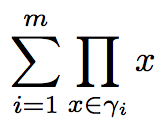# Expressing a sum of products as a fold

In an article I’m working on I’m talking about different execution orders for certain associative operations and the performance characteristics of each. At one place I talk about the following computationwhich is a sum of products. each $\gamma_i$ is a set of objects for which a product is defined. For the sake of this example, assume it is a set of objects of type Double. To compute this in Scala the most concise we that I have found is the following.

def sumOfProducts(seq: Seq[Seq[Double]]: Double = {
seq.map { gamma =>
gamma.fold(1.0)( _ * _)
}.fold(0.0)(_ + _)
}


However, it seems that I should be able to express this as a fold inside a fold, which would correspond closer to the mathematical notation. Can something think of a way to express this as just two fold operations, without the map?

The Scala code as three loops (one map, and two fold loops). However, the mathematical notation has only two loops. Or is there a 3rd loop in the mathematical notation that I don’t see?

you can replace the map with

seq.foldLeft(0.0) {
(sum, gamma) => sum + gamma.fold(0.0)(_ * _)
}


Thanks, but small correction, the inner identity should be 1.0, not 0.0, i.e., the identity for _*_.

It is curious why one conversion has 3 loops and the other just has two. But I think I understand now. The map based version uses map to accumulate a sequence to thereafter fold over. The concentric fold version doesn’t accumulate the sequence, but rather folds over it directly as it is being computed. My suspicion is that this is some sort of category-theoretical concept of tracing arrows through different paths to arrive at the same result.

I’d expect any category theoretical discussion of this to be way beyond my grasp.Just two remarks:

• The “concentric” variant using #foldLeft() is actually more general, since it allows for non-associative accumulation operations.
• Depending on the environment, the map/fold variant may be more efficient - e.g. with parallel collections(?) or in Spark.
1 Like